Advertising space available Members Area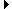Sign Up | Log In
 Free Level 2 QuotesStock AnalysisCorrelation Trading PlatformCorrelationsMembership Benefits

SMAMSPEED
 Lookup a different indicator.

 Indicator Usage Description Example smamspeed (smamspeed[x,y]) , where x is a positive integer and y {a,d,n,rd,ru}. Returns a 1 if the the acceleration of the slope of the x-day simple moving average matches y, a = Accelerating, d=Decelerating, n = Neutral, rd = Reversal Down, ru = Reversal Up (smamspeed[25,a]) and (smamdir[25,m])

 Introduction: The 'smamspeed' indicator is also known as the simple moving average slope speed. Smamspeed was created and developed by AiStockCharts.com. It is used to identify stocks where the acceleration of the slope of the simple moving average is accelerating, decelerating, neutral, reversed down or reversed up. This indicator is different than just identifying the slope of the moving average. Instead, this indicator identifies the rate of change of the slope. For example, the slope of the moving average can be positive yet have a neutral smamspeed. Example:The example in the table above is read as 'Find hits on stocks that had an accelerating negative sloped 25-day simple moving average.' The following stock chart of Home Depot highlights in yellow some hits on this example indicator. The sensitivity of the smamspeed indicator is set to detect at least a 0.1% change in the slope of the moving average.INDICATORS IN OUR DATABASE THAT INCLUDE THE SMAMSPEED INDICATOR...

Click here to view all indicators that include the indicator 'smamspeed' (sorted by AISCORE)

 (smamspeed[20,ru])AISCORE: 3157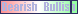Indicator ID: 051101195715(smamspeed[22,ru])AISCORE: 3332Indicator ID: 051106200729(smamspeed[21,ru])AISCORE: -567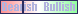Indicator ID: 051107114441(smamspeed[19,ru])AISCORE: 15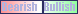Indicator ID: 051107114635(smamspeed[20,rd])AISCORE: 1425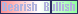Indicator ID: 051107220236 More ->